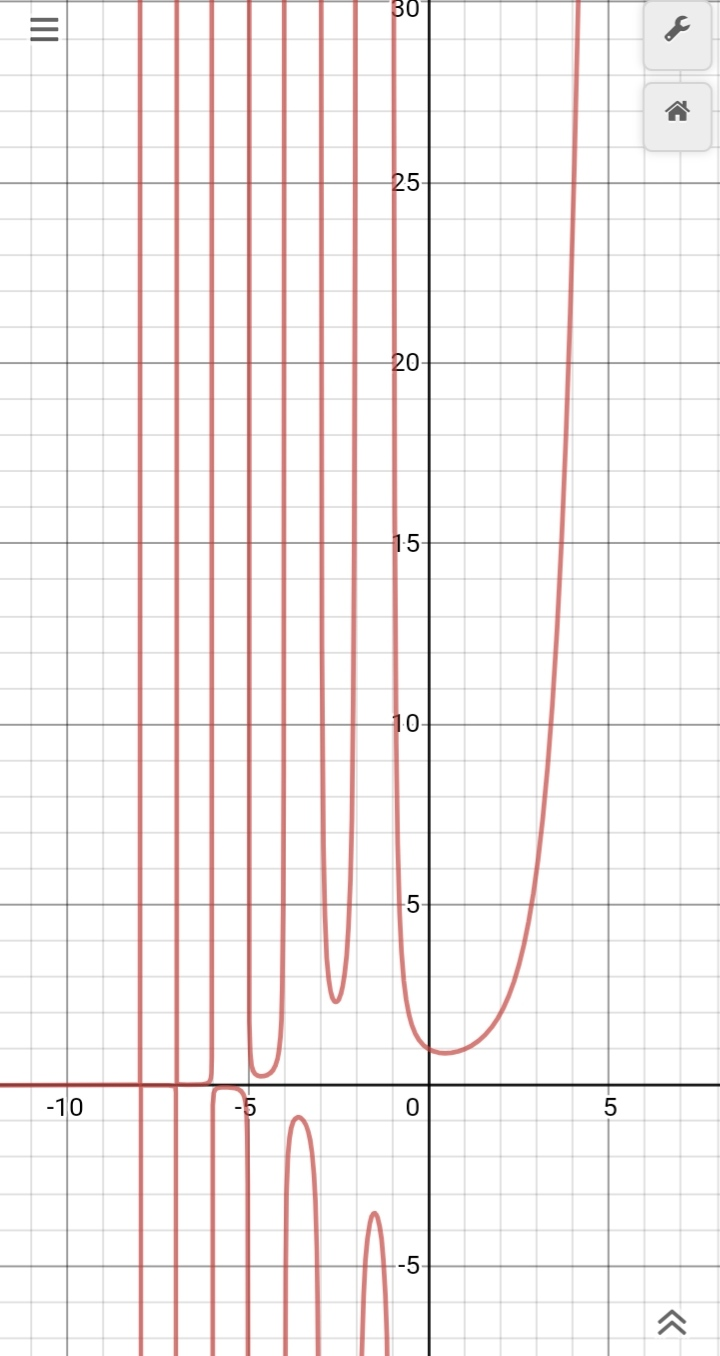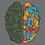# Expanding the factorial

What is the factorial of some $0 < x \leq 1$?

First what's a factorial? The factorial of a number n is the number of ways of arranging n objects in a line. Mathematically,

$n!=n(n-1)(n-2)(n-3)\dotsm(3)(2)(1)$

So \begin{aligned}1! &= 1 \\ 2! &= 2 \\ 3! &= 6 \\ 4! &=24 \\ \dots \text{ and so on.} \end{aligned}

So what is the value of $\dfrac{1}{2}!$?

To find the answer, let us first see the graph of $n!$Desmos

Surprise! Surprise!! Turns out, $n!$ exists for negative integers as well. How? For that, we return to the graph again.

We can see that the function satisfies -

\begin{aligned}f(1) &= 1 \\ f(x) &= xf(x)\end{aligned}

It is a "smooth" graph. So $\ln f(x)$ is convex.

Turns out, there's actually another function that satisfies all the three above conditions - the gamma function.

$\Gamma(x)=\int_0^\infty \mathrm{t}^{x-1}{e}^{-t},\mathrm{d}t$

This would converge for some values of x, for some values, it won't.

So this leads to $x!=\Gamma(x+1)$

We can now find the value of $\dfrac{1}{2}! = \dfrac{\sqrt\pi}{2}$

Importance of the gamma function-

It pops up in many applications like quantum physics, fluid dynamics, statistics, number theory.

Bohr-Mollerup theorem

Numerical computation

Alternative ways

Inspiration1 year, 5 months ago

This discussion board is a place to discuss our Daily Challenges and the math and science related to those challenges. Explanations are more than just a solution — they should explain the steps and thinking strategies that you used to obtain the solution. Comments should further the discussion of math and science.

When posting on Brilliant:

• Use the emojis to react to an explanation, whether you're congratulating a job well done , or just really confused .
• Ask specific questions about the challenge or the steps in somebody's explanation. Well-posed questions can add a lot to the discussion, but posting "I don't understand!" doesn't help anyone.
• Try to contribute something new to the discussion, whether it is an extension, generalization or other idea related to the challenge.

MarkdownAppears as
*italics* or _italics_ italics
**bold** or __bold__ bold
- bulleted- list
• bulleted
• list
1. numbered2. list
1. numbered
2. list
Note: you must add a full line of space before and after lists for them to show up correctly
paragraph 1paragraph 2

paragraph 1

paragraph 2

[example link](https://brilliant.org)example link
> This is a quote
This is a quote
    # I indented these lines
# 4 spaces, and now they show
# up as a code block.

print "hello world"
# I indented these lines
# 4 spaces, and now they show
# up as a code block.

print "hello world"
MathAppears as
Remember to wrap math in $$ ... $$ or $ ... $ to ensure proper formatting.
2 \times 3 $2 \times 3$
2^{34} $2^{34}$
a_{i-1} $a_{i-1}$
\frac{2}{3} $\frac{2}{3}$
\sqrt{2} $\sqrt{2}$
\sum_{i=1}^3 $\sum_{i=1}^3$
\sin \theta $\sin \theta$
\boxed{123} $\boxed{123}$

Sort by:

Really good! But calculators don't show it, why? @Adhiraj Dutta

- 1 year, 2 months ago

Because they don't use the gamma function. Instead they use an approximation using Stirling's approximation.

I found this on a reddit post.

- 1 year, 2 months ago

- 1 year, 2 months ago

I have a challenge for you - can you find $\frac{\pi}{4}$ $!$?

- 1 year, 1 month ago

Isn't it $\displaystyle \sum_{n = 0}^\infty \frac{\pi}{3((n+1)(n+3))} ?$ :P

- 1 year, 1 month ago

That's not what my question was about...

- 1 year, 1 month ago

Did you mean factorial of $\dfrac{\pi}{4}?$. Use Wolfram Alpha.

- 1 year, 1 month ago